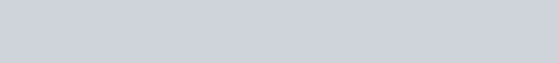Find the missing frequency p for the following frequency distribution whose mean is 28.25.
Question:

Find the missing frequency p for the following frequency distribution whose mean is 28.25.Solution:

We will prepare the following table:Thus, we have:

Mean $=\frac{\sum f_{i} x_{i}}{\sum x_{i}}$

$\Rightarrow 28.25=\frac{1445+25 p}{50+p}$

$\Rightarrow 28.25(50+p)=(1445+25 p)$

$\Rightarrow 1412.5+28.25 p=1445+25 p$

$\Rightarrow 3.25 p=32.5$

$\Rightarrow p=10$